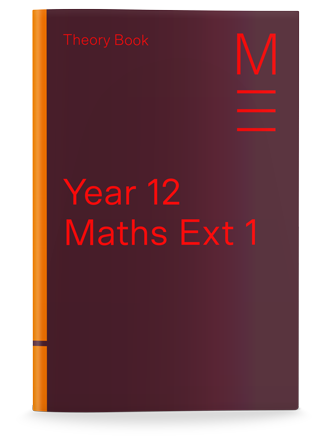Year 12 Maths Ext 1 Holiday Course

Get ahead in the school holidays

Gain an in-depth knowledge and understanding of the key math ext 1 topics over 2 weeks in the school holidays. During the term, you’ll have more time to practise maths exam questions.

Problem solving techniques

Learn problem-solving techniques that are not in your textbook. You’ll be able to solve unfamiliar and challenging maths ext 1 questions. Every time.

Working with confidence

Focus on balancing between speed and accuracy now that you have a greater understanding and confidence. With the best teachers, resources and support on your side there’s nothing to fear!

Year 12 Maths Ext 1 Holiday Course students receive

Mathematics Theory Book

Mathematics Work Book

Daily Mathematics Quiz

Mathematics Workshops

End of Course Topic TestGet ahead with our 9-day School Holiday Courses

Year 12 Maths Ext 1 Holiday Accelerated Course Program

Topics

Vectors

Revision of Differentiation

Integration

Mathematical Induction

Topics

Further Trigonometric Equations

Calculus of Trigonometric Functions

Calculus of Inverse Trigonometric Functions

Methods of Integration

Topics

Projectile Motion

Differential Equations

Further Statistics

Topics

Arithmetic and Geometric Progressions

Financial Mathematics

HSC Mark Booster

Year 12 Maths Ext 1 Holiday Accelerated Course Details

9 daily lessons in the school holidays

September - October

Vectors, Revision of Differentiation, Integration & Mathematical Induction

Lesson 1: Vectors 1

Lesson 2: Vectors 2

Lesson 3: Vectors 3

Lesson 4: Revision of Differentiation

Lesson 5: Integration 1

Lesson 6: Integration 2

Lesson 7: Integration 3

Lesson 8: Mathematical Induction

Lesson 9: Revision and Topic Test

January - January

Further Trigonometric Equations, Calculus of Trigonometric Functions, Calculus of Inverse Trigonometric Functions and Methods of Integration

Lesson 1: Calculus of Trigonometric Functions 1

Lesson 2: Calculus of Trigonometric Functions 2

Lesson 3: Calculus of Inverse Trigonometric Function 1

Lesson 4: Calculus of Inverse Trigonometric Function 2

Lesson 5: Methods of Integration

Lesson 6: Revision of Trigonometric Identities

Lesson 7: Further Trigonometric Equations 1

Lesson 8: Further Trigonometric Equations 2

Lesson 9: Revision and Topic Test

April - April

Projectile Motion, Differential Equations, Further Statistics

Lesson 1: Projectile Motion 1

Lesson 2: Projectile Motion 2

Lesson 3: Differential Equations 1

Lesson 4: Differential Equations 2

Lesson 5: Bivariate Data Analysis

Lesson 6: Continuous Random Variables

Lesson 7: The Normal Distribution

Lesson 8: The Binomial Distribution

Lesson 9 End of Term Topic Test

June - July

Arithmetic and Geometric Progressions, Financial Mathematics, HSC Mark Booster

Lesson 1: Arithmetic Progression and Applications

Lesson 2: Geometric Progression and Applications

Lesson 3: Financial Mathematics

Lesson 4: Review of Trigonometric Functions

Lesson 5: Proofs Involving the Binomial Theorem

Lesson 6: Permutations, Combinations and the Binomial Expansion

Lesson 7: Differential Equations and Curve Sketching

Lesson 8: Vectors and the Projectile Motion

Lesson 9: Mock HSC Exam

3 Hour Lesson Breakdown

Surprise yourself with how much you can learn in 3 hours

 30 min Introduction Revision, In-class Quiz and Overview Teachers revise previous lesson and answer homework questions before conducting an In-class Quiz. Teachers revise previous lesson and answer homework questions before conducting an In-class Quiz. 65 min Theory Lesson Part 1 Gain in-depth knowledge and understanding Teachers explain key mathematics concepts which are reinforced by completing exam-style questions. Teachers explain key mathematics concepts which are reinforced by completing exam-style questions. 10 min Break 65 min Theory Lesson Part 2 Gain in-depth knowledge and understanding Teachers explain key mathematics concepts which are reinforced by completing exam-style questions. Teachers explain key mathematics concepts which are reinforced by completing exam-style questions. 10 min Review Q&A Students ask questions to clarify understanding. Students ask questions to clarify understanding.

per hour

x

per lesson

x

per course

=

\$1248

per course (inc. GST)

What you'll get

• Additional online resources including practice papers and solutions

Love us or walk away!
All courses come with a First Lesson Money Back Guarantee.

Matrix COVID-19 Learning Guarantee

Enrol with confidence. If a Matrix 2022 Term 2 student is absent from their on campus lesson because they are unwell or in self-isolation due to COVID-19, Matrix will provide them access to their missed class with an online video lesson. Learn more here.

How is Matrix different?

Matrix OthersTeachers

Matrix

Vast classroom teaching experience, HSC and/or university teaching experience with an ability to explain concepts clearly

Others

Have little or no actual teaching experience, cannot explain difficult concepts in a manner that students can understandProgram

Matrix

Teaching program that covers the NSW Education Standards Authority syllabus. Structured learning system that allows students to develop sound study habits every week

Others

Most private tutors and other tuition centres do not follow a set structure; rather they have an ad-hoc approach without considering timing and outcomesResources

Matrix

A full set of resources written by academics and education researchers. Online access to Supplementary exam papers / texts with top responses.

Others

Only address content that students raise, use generic textbooks.Assessments

Matrix

Short quizzes and topic tests held under strict exam conditions to ensure students have a solid understanding of the subject

Others

No exam-style quizzes or tests that assess the student’s weaknesses. Hence, they are not able to identify their specific learning needsReporting

Matrix

Weekly tracking of Grades, Quiz and Topic Test results so student and parents can monitor their progress.

Others

No measured tracking process, so they cannot tell if the student is struggling or improving.Learning Management System

Matrix

Share information, academic resources and advice with classmates from over 220 schools.

Others

Do not have the library of resources or past exams that can help students excelOne-to-one Workshops

Matrix

Free workshops to target individual learning needs

Others

No additional support outside the lesson.